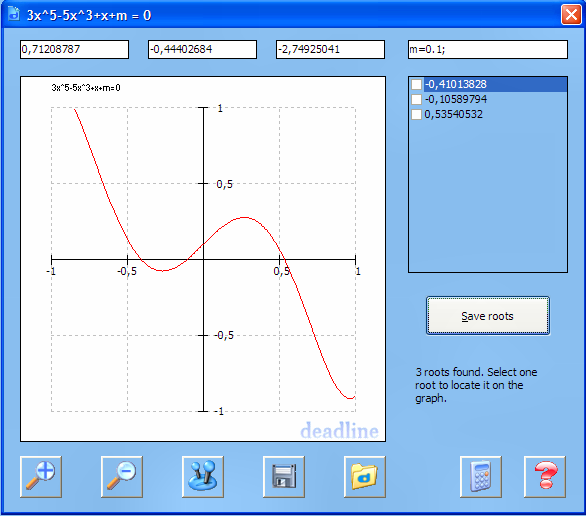Freeware

• ##### VIRUS REPORTS

100%Clean

virus check results

0/10

• ##### RUNS ON

Win98,WinME,WinNT 4.x,Windows2000,WinXP,Windows2003,Win Vista

1.39MB

12-May-2007

• ##### DEVELOPER

Ionut Alex. Chitu

##### Authors Description

DeadLine is a free program useful for solving equations, plotting graphs and obtaining an in-depth analysis of a function. Designed especially for students and engineers, the freeware combines graph plotting with advanced numerical calculus, in a very intuitive approach. Most equations are supported, including algebraic equations, trigonometric equations, exponential equations, parametric equations. DeadLine solves equations graphically and numerically. It displays the graph of the function and a list of the real roots of the equation. You can evaluate the function and the first two derivatives, find extrema of the function and integrate numerically. You can even obtain the first two derivatives. Save the results provided by DeadLine so you can easily integrate in your school projects. While there is no flawless method for solving equations, the program combines the most successful methods in order to deliver you the right answer. No more problems on getting your homework done. Meet the DeadLine, the free equation solver.

••